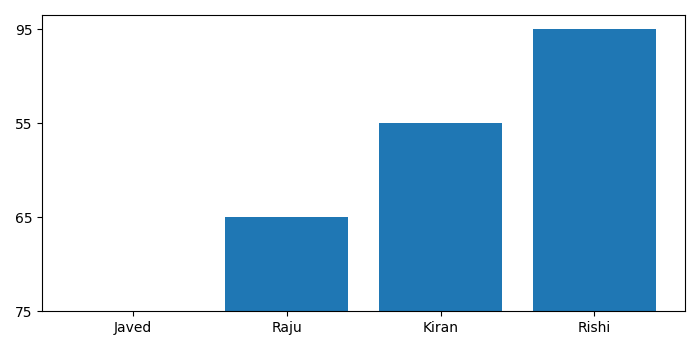# How to plot a very simple bar chart (Python, Matplotlib) using input *.txt file?

MatplotlibPythonData Visualization

To plot a very simple bar chart from an input text file, we can take the following steps −

• Make an empty list for bar names and heights.

• Read a text file and iterate each line.

• Append names and heights into lists.

• Plot the bar using lists (Step 1).

• To display the figure, use show() method.

## Example

from matplotlib import pyplot as plt
plt.rcParams["figure.figsize"] = [7.00, 3.50]
plt.rcParams["figure.autolayout"] = True
bar_names = []
bar_heights = []
for line in open("test_data.txt", "r"):
bar_name, bar_height = line.split()
bar_names.append(bar_name)
bar_heights.append(bar_height)
plt.bar(bar_names, bar_heights)
plt.show()

"test_data.txt" contains the following data −

Javed 75
Raju 65
Kiran 55
Rishi 95

## Output